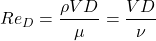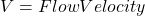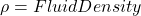# Hydraulic Diameter – Purpose, Formula, Alternatives, Applications

#### Engineering

Hydraulic diameter, DH, is the characteristic length used to determine Reynolds number when dealing with flow in non-circular mediums. Reynolds number may then be of use in determining whether a flow is laminar or turbulent. Similarly, it may help in other fluid flow rate calculations and in determining pressure drop across channels. In this article, you will learn the purpose of hydraulic diameter, its formula, real-world applications, and alternatives.

## The Purpose of Hydraulic Diameter

Hydraulic diameter provides an alternative to which non-circular piping may be likened to circular ones to determine various characteristics of a fluid flow. Determining the value is different for various geometries. While diameter considerations are best for turbulent flows, it is best to avoid it for laminar flows where the shape of the piping plays a larger role on the flow. Additionally, it is mainly of use in calculations of turbulent flows it helps in finding shear stresses associated with the flow in question. It is also of use in heat transfer calculations that consider internal flow.

## Hydraulic Diameter Formulas

The calculations stem off of the basic equation below:Where:Hydraulic diameter is a component in calculating Reynolds number. Reynolds number is of use to determine the nature of a viscous flow, whether it is laminar or turbulent. Reynolds number is a crucial parameter to consider when designing systems that deal with viscous flow. The formula below shows how it relates to the calculation of Reynolds number.Where:Working off the principles of the basic equation, formulas are derived for a variety of different shapes. Most of the major ones are as follows:

## Alternative Measurements

Other types of measurements closely relate to hydraulic diameter. These are hydraulic radius, equivalent diameter, characteristic length, and pressure drop.

Hydraulic radius sees more frequent use than the hydraulic diameter for open channel flows. It is defined as the cross-sectional area of flow over the wetted perimeter. Relationally speaking, it may be understood as a function of hydraulic radius. It is not twice the value of the hydraulic radius but four times the amount.### Equivalent Diameter

Equivalent diameter is a term that refers to the diameter that gives way to the same pressure loss as a set rectangular pipe or channel. As the two terms are not related because the equivalent diameter is dependent on the value of an existing pipe’s pressure loss. Additionally, equivalent diameter is important to consider if switching of piping design or shape becomes necessary in an operating facility.

### Characteristic Length

In terms of fluid flow, characteristic length refers to the ratio of volume in the body to the surface area. Characteristic length is substituted for Hydraulic Diameter in cases where the pipe or duct in question is circular. In conclusion, characteristic length is of use in cases of circular flow channels and hydraulic diameter for other shapes.

### Pressure Drop

In terms of fully laminar flow, pressure drop per unit of length for an incompressible fluid varies through an inverse relationship with the Reynolds number. Additionally, the Reynolds number is determined by the hydraulic diameter of a piping system, it too varies inversely with pressure drop in this case.